Electron. J. Differential Equations, Vol. 2018 (2018), No. 156, pp. 1-17.

### Fractional p-Laplacian equations on Riemannian manifolds Lifeng Guo, Binlin Zhang, Yadong Zhang

Abstract:
In this article we establish the theory of fractional Sobolev spaces on Riemannian manifolds. As a consequence we investigate some important properties, such as the reflexivity, separability, the embedding theorem and so on. As an application, we consider fractional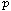-Laplacian equations with homogeneous Dirichlet boundary conditions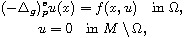where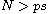with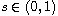,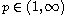,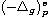is the fractional p-Laplacian on Riemannian manifolds, (M,g) is a compact Riemannian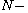manifold,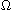is an open bounded subset of M with smooth boundary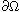, and f is a Caratheodory function satisfying the Ambrosetti-Rabinowitz type condition. By using variational methods, we obtain the existence of nontrivial weak solutions when the nonlinearity f satisfies sub-linear or super-linear growth conditions.

Submitted August 18, 2017. Published August 22, 2018.
Math Subject Classifications: 35R01, 35R11, 35A15.
Key Words: Fractional p-Laplacian; Riemannian manifolds; variational methods.

Show me the PDF file (298 KB), TEX file for this article.Lifeng Guo School of Mathematics and Statistics Northeast Petroleum University Daqing 163318, China email: lfguo1981@126.com Binlin Zhang Department of Mathematics Heilongjiang Institute of Technology Harbin 150050, China email: zhangbinlin2012@outlook.com Yadong Zhang School of Mathematics and Statistics Northeast Petroleum University Daqing 163318, China email: chichidong@foxmail.com